## If a snowball melts so that its surface area decreases at a rate of 8 cm2/min, find the rate (in cm/min) at which the diameter decreases whe

Question

If a snowball melts so that its surface area decreases at a rate of 8 cm2/min, find the rate (in cm/min) at which the diameter decreases when the diameter is 11 cm. (Round your answer to three decimal places.)

in progress 0
6 months 2021-07-16T21:07:59+00:00 1 Answers 4 views 0

## Answers ( )

Step-by-step explanation:

I’m going to go through this as I would when teaching it to my students for the first time. This is a super simple one, so it shouldn’t be too hard. Begin with what you know:

A snowball is a sphere. Since we are told that the surface area is changing (decreasing, to be exact), it would make sense to know the formula for the surface area of a sphere: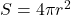where r is the radius. Let’s take the derivative of this using implicit differentiation to see what it is we need to solve this: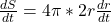, where dr/dt is the rate at which the radius is changing. If we look at the problem, we are looking for the rate at which the DIAMETER is changing. So instead of using the radius in the original formula, we need to write it in terms of the diameter. We know that

d = 2r so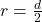and we will put that into the formula for r to get: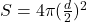and simplify a bit: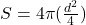and the 4’s cancel each other out, leaving us with simply: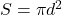Now let’s take the derivative: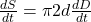where the rate of the surface area is -8 (“decreasing” rate makes this number a negative) when the diameter is 11. Filling in: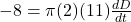and solving for the rate at which the diameter is changing: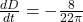, which rounds to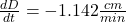and again, the negative means that the diameter is decreasing.